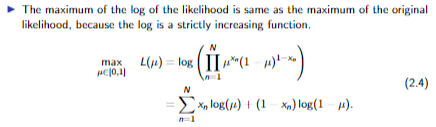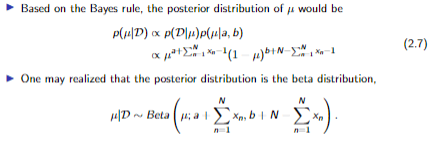# Bernoulli and Bayesian probabilities

Summary:: Hello there, I'm a mechanical engineer pursuing my graduate degree and I'm taking a class on machine learning. Coding is a skill of mine, but statistics is not... anyway, I have a homework problem on Bernoulli and Bayesian probabilities. I believe I've done the first few parts correctly, but the final question asks me to explain why one is more accurate than another, and the inverse as well. I am not sure, so I figured I'd reach out here and ask. The work and appropriate equations are below:

1. (10 pts) Consider 20 values randomly sampled from the Bernoulli Distribution with parameter :

Matlab:
x = [1, 1, 0, 1, 1, 0, 0, 1, 1, 1, 0, 1, 1, 1, 1, 0, 1, 1, 1, 1];
N = length(x);

(a) Estimate the parameter using the maximum likelihood approach and the 20 data values.
Matlab:
u = sum(x==1)/N; % u = 0.75
bern = (u.^x).*(1-u).^(1-x)

p = 0;
for n = 1:N
pTemp = x(n)*log(u) + (1-x(n))*log(1-u);
p = p+pTemp;
end

%ln(a) = b <--> a = e^b
p = exp(p); % p = 1.3050e-05
(b) Estimate the parameter using the Bayesian approach. Use the beta distribution Beta(a=8, b=4).
Matlab:
% a + sum(xn),b + N - sum(xn)
% (8 + 15 - 1) / (12 + 20 - 2) = 22/30
u = 22/30; % u = 0.7333

(c) Estimate the parameter using the Bayesian approach. Use the beta distribution Beta(a=4, b=8).
Matlab:
% (4 + 15 - 1) / (12 + 20 - 2) = 18/30
u = 18/30; % u = 0.6
(d) Discuss why the estimation from (b) is more accurate than that from (a) and why the estimation from (c) is worse than that from (a).
Matlab:
uA = 0.75;
uB = 0.7333;
uC = 0.6;Thanks in advance for any help!!

andrewkirk
Homework Helper
Gold Member
I think something goes wrong in the first step (a).
The max likelihood estimate of the Bernoulli parameter is simply the number of 1s divided by the sample size, which gives 15/20 = 0.75.
I don't understand your reason for doing the calcs you show above, which appear to give an answer of approx 10^-5.

It is too hard to work out what you were trying to do based only on computer code. Better to write out mathematical reasoning and explain the steps you took.

Also, we can't assess accuracy without knowing what the population parameter is. It looks like you meant to include that in the problem statement, but it is missing.

•hdp12
I did a little too much in part A, you're right.

nothing else was provided in the problem statement though. Is there any reason why one estimation would be more accurate than another method of estimation?

ChrisVer
Gold Member
Do you know the true value of your parameter u (e.g. with what value of the parameter your x was generated)? Otherwise I don't understand the question about accuracy ... How would you know if the MLE or the Bayesian posterior is more accurate if you don't know the actual value that you try to predict in this case?

However, if I assume that true value should be somewhere near 0.7, then the comparison between (c)-(a) is rather straightforward if you plot your Beta function prior in the case of (c)...

andrewkirk